Select Page

Of all the mathematical theorems, postulates and conjectures, the Fibonacci sequence stands out as the most useful modern tool devised by the ancient mathematicians. Its simplicity makes it accessible to the student or layman and its application in finance or search algorithms gives it credence among exceedingly complex calculations. It is also a romantic notion, easily repeated like a popular song with a great history. Like Pythagoras or Euclid, Leonardo “Fibonacci” di Pisa brought complex mathematics into popular knowledge and he has been repaid by the enormous use of his product.

Fibonacci was a merchant and mathematician. He was born in the twelfth century and his contributions to science affect all of us. His most underrated work may be adapting Hindu-Arabic numerals, zeros and decimal places and presenting them to European colleagues in his book, Liber Abaci (Sigler, Laurence E. [trans.] 2002). When we are driving, these numbers are used to measure speed or the distance to the next freeway exit. At work or shopping, numbers reflect material gains or the cost of food or merchandise. As a result of increased competition in sporting events, digital clocks with multiple decimal places are required to pick the winner. We may be oblivious to this when we are fast asleep but the moment our eyes crack open, a clock with numbers may be staring back at us.

While numbers are particularly important for definition throughout our lives, Fibonacci became a mathematical superstar for a simple sequence of numbers that appears in Liber Abaci. The sequence is defined as: Beginning with 0 then 1, each subsequent number is the sum of the previous two. As illustrated below, the sequence uses a simple formula and real numbers to recreate a growth system that approximates the golden ratio (Table 1), defined by: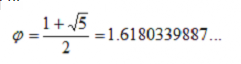Latin for Free Abacus, Liber Abaci was written to free tradesmen from esoteric calculations and computing machines. The abacus first saw light around 2500 B.C. and is still being used in parts of Asia and Africa. The abacus that Fibonacci may have used was comprised of a grooved board and a bunch of stones that were moved around to calculate addition, subtraction, multiplication, etc.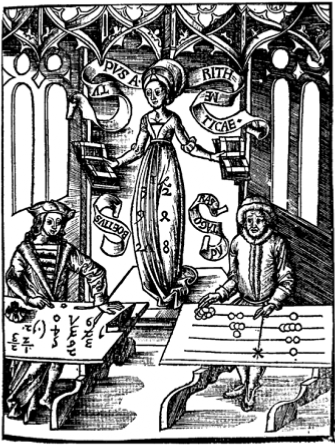The abacus at work Gregor Reisch, Margarita Philosophica, 1508 From Wikipedia

Fibonacci saw the abacus as ungainly and he championed written numerals as the solution. He used the Fibonacci sequence as proof that numerals could be applied to simple commerce and to the complexities of higher math and nature reflected by the golden ratio.

The golden ratio came from a time when market transactions were frequently done with a notched stick or knotted rope for storing numbers. Only scholars or higher degree tradesmen were familiar with calculating numbers or geometry. The golden ratio was used by stone cutters and sculptors as a modular template to create the exact proportions required for stone buildings or accurate artwork.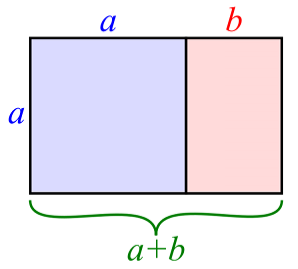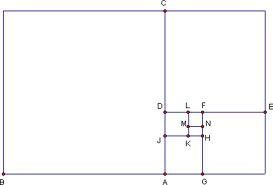Above: A graphical representation of the golden ratio. A square is laid next to a rectangle and a rectangle is formed with dimensions the same ratio as the first rectangle (images from Google search for “golden rectangle images”)

The Greek artist Phidias applied the ratio to his lifelike sculptures that stand in the Parthenon. This was the time of Euclid who first presented the golden ratio in Elements(Sir Thomas Heath[Trans]2006), written around 300 B.C. Before Fibonacci, most mathematical equations were performed by scratching in the dirt with a stick and complex math or logic was represented by geometric shapes, architecture and sculpture. These are not methods that can be universally applied in the 21st century.

Why would we consider these two methods when there are infinite options in modern mathematics using computers? A quick answer would be that time and computing power cost money. Business decisions are always mitigated by cost. The processes we employ on home computers, such as search algorithms, take time away from other pursuits. When we consider that most of the tasks we perform in our daily lives revolve around putting food on the table or improving our surroundings, there is little difference between us and the ancient cultures. Why not choose a method, exhaustively tested throughout the ages?

The figures below illustrate a worst case search for a 1×1 square in a given rectangle. This answers the question: How long will it take if the object is in the last place we look?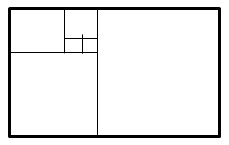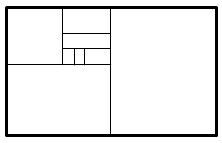The rectangle on the left represents a Fibonacci or golden ratio search. For a 13 x 8 rectangle, the search would take five steps using the Fibonacci numbers. The results of the golden ratio and Fibonacci numbers are almost identical. The difference is that a Fibonacci search needs a definite end point and the golden ratio can be used for unknown sequences or data. The rectangle on the right represents a binary search wherein the field is halved until a 1×1 block is reached. The difference is that the binary search takes two more steps to achieve the same result. For larger searches, the difference becomes exponential (table 2).

The next illustration is an example of Fibonacci numbers used by stock market analysts. Percentage values of 100%, 61.8%, 50%, 38.2% and 0% are used to predict movement after a large change in stock values. The golden ratio is represented by 61.8%(.618…), 38.2% is the remainder and 50% is a coin toss to mitigate the prediction. These numbers often coincide with areas of support(holding or buying) or resistance (holding or selling) caused by human interaction in the markets.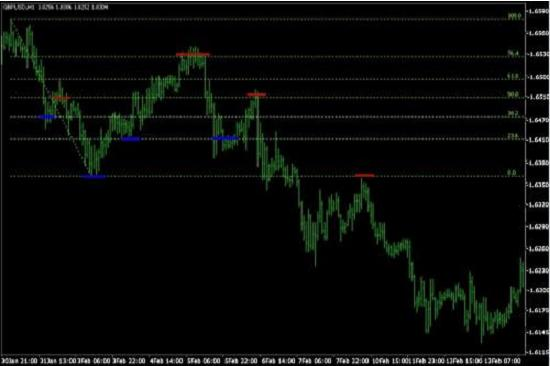Many prominent mathematicians and scientists are associated with the Fibonacci numbers or the golden ratio. Johannes Kepler (1571-1630), whose laws of planetary motion were furthered by Newton, proved the limit of Fibonacci numbers approached the golden ratio. Charles Bonnet (1720-1793) who discovered that caterpillars and butterflies “breathe” through their pores, pointed out that the spiral phylotaxis of plants were frequently two consecutive Fibonacci numbers. Plato and Pythagoras help fill out the list. Plato, a follower of Pythagoras described the Platonic solids in his book, Timaeus. Some of these geometric figures have relations with the golden ratio. Kepler used the golden ratio and the Pythagorean Theorem to create Kepler’s Triangle. With so many prominent figures in the mix, we can expect to see more of them in the future.

After exploring the wealth of information on the subject, we shouldn’t be surprised to find the Fibonacci sequence being used daily, right under our noses. Originally used to exemplify the use of written numbers, it is now used in the stock market to predict the growth of a company or product. It is also used as a basis for search algorithms that rival the speed of a binary search for small searches and the complexity of the golden ratio for large ones. From the ancient fields and markets, to modern day board rooms and class rooms, the Fibonacci sequence finds its place as a useful tool in the language of mathematics

References:

Sigler, Laurence E. (trans.) (2002), Fibonacci’s Liber Abaci, Springer-Verlag, ISBN 0-387-95419-8.

The Elements: Books I-XIII-Complete and Unabridged, (2006) Translated by Sir Thomas Heath, Barnes & Noble ISBN 0-7607-6312-7.

Numerical Methods Using Matlab, 4th Edition, 2004 John H. Mathews and Kurtis K. Fink ISBN: 0-13-065248-2http://math.fullerton.edu/mathews/n2003/fibonaccisearch/FibonacciSearchProof.pdf

Further resources: